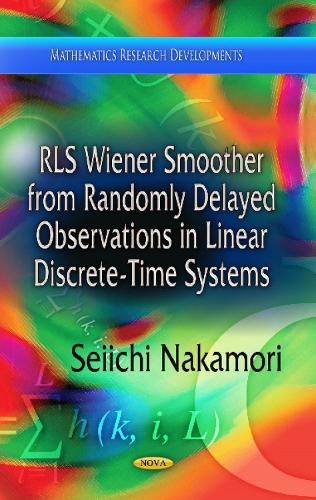•# RLS Wiener Smoother from Randomly Delayed Observations in Linear Discrete-Time Systems (Hardback)

(editor)
£104.99
Hardback 117 Pages / Published: 19/06/2013
• We can order this

Usually dispatched within 3 weeks

In this book, the new recursive least-squares (RLS) Wiener filter and fixed-point smoother are designed from randomly delayed observed values by one sampling time in linear discrete-time stochastic systems. The probability is given as a function of time. If the conditional probability is not a function of time, the length of the derivation for the RLS Wiener estimators becomes shorter than the current RLS Wiener algorithms for the fixed-point smoothing and filtering estimates. The proof for deriving the RLS Wiener fixed-point smoother and filter is shown in the case of the conditional probability as a function of time k. A numerical simulation example in Chapter 4 shows that the fixed-point smoothing and filtering algorithms, proposed in this book, are feasible. The RLS Wiener estimators do not use the information of the variance of the input noise and the input matrix in the state equation, in comparison with the estimation technique by the Kalman filter. Hence, the RLS Wiener estimation technique has an advantage that the estimation accuracy of the RLS Wiener estimators is not influenced by the estimation errors for the input noise variance and the input matrix.

Publisher: Nova Science Publishers Inc
ISBN: 9781624178184
Number of pages: 117
Weight: 278 g
Dimensions: 260 x 180 mm

£8.99   £7.99
Paperback
£3.95   £2.99
Paperback
£3.95   £2.99
Paperback
£8.99   £7.99
Paperback